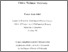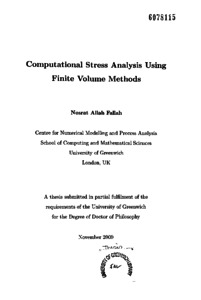# Computational stress analysis using finite volume methods

Fallah, Nosrat Allah (2000) Computational stress analysis using finite volume methods. PhD thesis, University of Greenwich.Preview
PDF (Pages containing signatures redacted)
Nosrat Allah Fallah 2000 - redacted.pdf - Published Version

## Abstract

There is a growing interest in applying finite volume methods to model solid mechanics problems and multi-physics phenomena. During the last ten years an increasing amount of activity has taken place in this area. Unlike the finite element formulation, which generally involves volume integrals, the finite volume formulation transfers volume integrals to surface integrals using the divergence theorem. This transformation for convection and diffusion terms in the governing equations, ensures conservation at the local element level. This is seen as a major attraction for finite volume methods.

The research presented in this thesis details the development of a cell vertex based finite volume formulation for complex analysis like geometrically nonlinear modelling and plate analysis. For both geometrically nonlinear and plate analysis a series of simulation results are presented and are compared with conventional finite element results.

Further research has been carried out to solve stress problems in multi-physics phenomena using a Computational Fluid Dynamics(CFD) frame-work. This approach has the advantage in that it uses the similarities between fluid and solid momentum equations to introduce some modifications in a CFD code that allows a complete CFD solution procedure to be used for the simultaneous calculation of the velocity, temperature and displacement variables. The results of this integrated approach are compared with results obtained by using techniques which solve the problem by 111 using two solvers (one for solid regions, one for fluid regions).

In summary, the novelty of the research detailed in this thesis is:

• Finite volulne formulation for elastic large strain analysis. Comparison of this approach with traditional finite element techniques:
- Cell-vertex finite volume method is as accurate as finite element approach but slower in solution time.

• Finite volume formulation for structural plate analysis. Comparison with traditional finite element method:
- Novel finite volume approach is as accurate as finite element approach.
- Does not display locking problems (observed with finite element methods) and is comparable in solution times.

• Formulation of an integrated CFD solver for coupled flow, heat transfer and stress calculations. Comparison with a 2-solver approach:
- Integrated approach is much faster and substantially less memory intensive than 2-solver approach.

Comparisons between the new formulation and traditional approaches are made in terms of accuracy and solution speed.

Item Type: Thesis (PhD) uk.bl.ethos.327338 stress analysis, calculation methods, numerical solution, dynamics, solid state physics, fluid mechanics, CFD, business data processing, Q Science > QC PhysicsT Technology > TA Engineering (General). Civil engineering (General) School of Computing & Mathematical SciencesSchool of Computing & Mathematical Sciences > Centre for Numerical Modelling & Process Analysis 25 Sep 2018 11:31 None None None None http://gala.gre.ac.uk/id/eprint/6166View Item